# Tag: non-commutative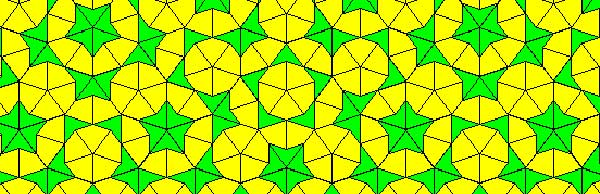Penrose tilings are aperiodic tilings of the plane, made from 2 sort of tiles : kites and darts. It is well known (see for example the standard textbook tilings and patterns section 10.5) that one can describe a Penrose tiling around a given point in the plane as an infinite sequence of 0’s and 1’s, subject to the condition that no two consecutive 1’s appear in the sequence. Conversely, any such sequence is the sequence of a Penrose tiling together with a point. Moreover, if two such sequences are eventually the same (that is, they only differ in the first so many terms) then these sequences belong to two points in the same tiling,

Another remarkable feature of Penrose tilings is their local isomorphism : fix a finite region around a point in one tiling, then in any other Penrose tiling one can find a point having an isomorphic region around it. For this reason, the space of all Penrose tilings has horrible topological properties (all points lie in each others closure) and is therefore a prime test-example for the techniques of noncommutative geometry.

In his old testament, Noncommutative Geometry, Alain Connes associates to this space a $C^*$-algebra $Fib$ (because it is constructed from the Fibonacci series $F_0,F_1,F_2,…$) which is the direct limit of sums of two full matrix-algebras $S_n$, with connecting morphisms

$S_n = M_{F_n}(\mathbb{C}) \oplus M_{F_{n-1}}(\mathbb{C}) \rightarrow S_{n+1} = M_{F_{n+1}}(\mathbb{C}) \oplus M_{F_n}(\mathbb{C}) \qquad (a,b) \mapsto ( \begin{matrix} a & 0 \\ 0 & b \end{matrix}, a)$

As such $Fib$ is an AF-algebra (for approximately finite) and hence formally smooth. That is, $Fib$ would be the coordinate ring of a smooth variety in the noncommutative sense, if only $Fib$ were finitely generated. However, $Fib$ is far from finitely generated and has other undesirable properties (at least for a noncommutative algebraic geometer) such as being simple and hence in particular $Fib$ has no finite dimensional representations…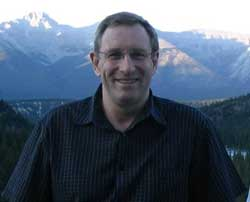A couple of weeks ago, Paul Smith discovered a surprising connection between the noncommutative space of Penrose tilings and an affine algebra in the paper The space of Penrose tilings and the non-commutative curve with homogeneous coordinate ring $\mathbb{C} \langle x,y \rangle/(y^2)$.

Giving $x$ and $y$ degree 1, the algebra $P = \mathbb{C} \langle x,y \rangle/(y^2)$ is obviously graded and noncommutative projective algebraic geometers like to associate to such algebras their ‘proj’ which is the quotient category of the category of all graded modules in which two objects become isomorphisc iff their ‘tails’ (that is forgetting the first few homogeneous components) are isomorphic.

The first type of objects NAGers try to describe are the point modules, which correspond to graded modules in which every homogeneous component is 1-dimensional, that is, they are of the form

$\mathbb{C} e_0 \oplus \mathbb{C} e_1 \oplus \mathbb{C} e_2 \oplus \cdots \oplus \mathbb{C} e_n \oplus \mathbb{C} e_{n+1} \oplus \cdots$

with $e_i$ an element of degree $i$. The reason for this is that point-modules correspond to the points of the (usual, commutative) projective variety when the affine graded algebra is commutative.

Now, assume that a Penrose tiling has been given by a sequence of 0’s and 1’s, say $(z_0,z_1,z_2,\cdots)$, then it is easy to associate to it a graded vectorspace with action given by

$x.e_i = e_{i+1}$ and $y.e_i = z_i e_{i+1}$

Because the sequence has no two consecutive ones, it is clear that this defines a graded module for the algebra $P$ and determines a point module in $\pmb{proj}(P)$. By the equivalence relation on Penrose sequences and the tails-equivalence on graded modules it follows that two sequences define the same Penrose tiling if and only if they determine the same point module in $\pmb{proj}(P)$. Phrased differently, the noncommutative space of Penrose tilings embeds in $\pmb{proj}(P)$ as a subset of the point-modules for $P$.

The only such point-module invariant under the shift-functor is the one corresponding to the 0-sequence, that is, corresponds to the cartwheel tiling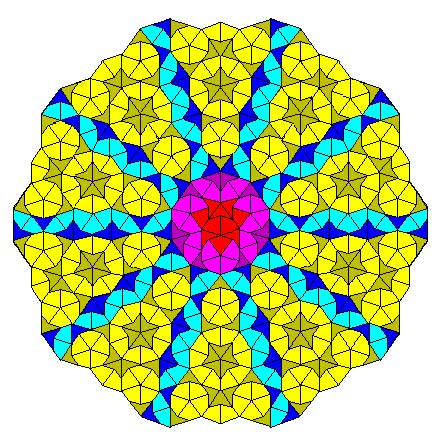Another nice consequence is that we can now explain the local isomorphism property of Penrose tilings geometrically as a consequence of the fact that the $Ext^1$ between any two such point-modules is non-zero, that is, these noncommutative points lie ‘infinitely close’ to each other.

This is the easy part of Paul’s paper.

The truly, truly amazing part is that he is able to recover Connes’ AF-algebra $Fib$ from $\pmb{proj}(P)$ as the algebra of global sections! More precisely, he proves that there is an equivalence of categories between $\pmb{proj}(P)$ and the category of all $Fib$-modules $\pmb{mod}(Fib)$!

In other words, the noncommutative projective scheme $\pmb{proj}(P)$ is actually isomorphic to an affine scheme and as its coordinate ring is formally smooth $\pmb{proj}(P)$ is a noncommutative smooth variety. It would be interesting to construct more such examples of interesting AF-algebras appearing as local rings of sections of proj-es of affine graded algebras.

In view or recents events & comments, some changes have been made or will be made shortly :

categories : Sanitized the plethora of wordpress-categories to which posts belong. At the moment there are just 5 categories : ‘stories’ and ‘web’ (for all posts with low math-content) and three categories ‘level1’, ‘level2’ and ‘level3’, loosely indicating the math-difficulty of a post.

MathJax : After years of using LatexRender and WP-Latex, we’ll change to MathJax from now on. I’ll try to convert older posts as soon as possible. (Update : did a global search and replace. ‘Most’ LaTeX works, major exceptions being matrices and xymatrix commands. I’ll try to fix those later with LatexRender.)

theme : The next couple of days, the layout of this site may change randomly as I’ll be trying out things with the Swift wordpress theme. Hopefully, this will converge to a new design by next week.

name : Neverendingbooks will be renamed to something more math-related. Clearly, the new name will depend on the topics to be covered. On the main index page a pop-up poll will appear in the lower right-hand corner after 10 seconds. Please fill in the topics you’d like us to cover (no name or email required).

This poll will close on friday 21st at 12 CET and its outcome will influence name/direction of this blog. Use it also if you have a killer newname-suggestion. Among the responses so far, a funnier one : “An intro to, or motivation for non-commutative geometry, aimed at undergraduates. As a rule, I’d take what you think would be just right for undergrads, and then trim it down a little more.”

guest-posts : If you’d like to be a guest-blogger here at irregular times, please contact me. The first guest-post will be on noncommutative topology and the interpretation of quantum physics, and will appear soon. So, stay tuned…

This is a belated response to a Math-Overflow exchange between Thomas Riepe and Chandan Singh Dalawat asking for a possible connection between Connes’ noncommutative geometry approach to the Riemann hypothesis and the Langlands program.

Here’s the punchline : a large chunk of the Connes-Marcolli book Noncommutative Geometry, Quantum Fields and Motives can be read as an exploration of the noncommutative boundary to the Langlands program (at least for $GL_1$ and $GL_2$ over the rationals $\mathbb{Q}$).

Recall that Langlands for $GL_1$ over the rationals is the correspondence, given by the Artin reciprocity law, between on the one hand the abelianized absolute Galois group

$Gal(\overline{\mathbb{Q}}/\mathbb{Q})^{ab} = Gal(\mathbb{Q}(\mu_{\infty})/\mathbb{Q}) \simeq \hat{\mathbb{Z}}^*$

and on the other hand the connected components of the idele classes

$\mathbb{A}^{\ast}_{\mathbb{Q}}/\mathbb{Q}^{\ast} = \mathbb{R}^{\ast}_{+} \times \hat{\mathbb{Z}}^{\ast}$

The locally compact Abelian group of idele classes can be viewed as the nice locus of the horrible quotient space of adele classes $\mathbb{A}_{\mathbb{Q}}/\mathbb{Q}^{\ast}$. There is a well-defined map

$\mathbb{A}_{\mathbb{Q}}’/\mathbb{Q}^{\ast} \rightarrow \mathbb{R}_{+} \qquad (x_{\infty},x_2,x_3,\ldots) \mapsto | x_{\infty} | \prod | x_p |_p$

from the subset $\mathbb{A}_{\mathbb{Q}}’$ consisting of adeles of which almost all terms belong to $\mathbb{Z}_p^{\ast}$. The inverse image of this map over $\mathbb{R}_+^{\ast}$ are precisely the idele classes $\mathbb{A}^{\ast}_{\mathbb{Q}}/\mathbb{Q}^{\ast}$. In this way one can view the adele classes as a closure, or ‘compactification’, of the idele classes.

This is somewhat reminiscent of extending the nice action of the modular group on the upper-half plane to its badly behaved action on the boundary as in the Manin-Marcolli cave post.

The topological properties of the fiber over zero, and indeed of the total space of adele classes, are horrible in the sense that the discrete group $\mathbb{Q}^*$ acts ergodically on it, due to the irrationality of $log(p_1)/log(p_2)$ for primes $p_i$. All this is explained well (in the semi-local case, that is using $\mathbb{A}_Q’$ above) in the Connes-Marcolli book (section 2.7).

In much the same spirit as non-free actions of reductive groups on algebraic varieties are best handled using stacks, such ergodic actions are best handled by the tools of noncommutative geometry. That is, one tries to get at the geometry of $\mathbb{A}_{\mathbb{Q}}/\mathbb{Q}^{\ast}$ by studying an associated non-commutative algebra, the skew-ring extension of the group-ring of the adeles by the action of $\mathbb{Q}^*$ on it. This algebra is known to be Morita equivalent to the Bost-Connes algebra which is the algebra featuring in Connes’ approach to the Riemann hypothesis.

It shouldn’t thus come as a major surprise that one is able to recover the other side of the Langlands correspondence, that is the Galois group $Gal(\mathbb{Q}(\mu_{\infty})/\mathbb{Q})$, from the Bost-Connes algebra as the symmetries of certain states.

In a similar vein one can read the Connes-Marcolli $GL_2$-system (section 3.7 of their book) as an exploration of the noncommutative closure of the Langlands-space $GL_2(\mathbb{A}_{\mathbb{Q}})/GL_2(\mathbb{Q})$.

At the moment I’m running a master-seminar noncommutative geometry trying to explain this connection in detail. But, we’re still in the early phases, struggling with the topology of ideles and adeles, reciprocity laws, L-functions and the lot. Still, if someone is interested I might attempt to post some lecture notes here.

The general public expects pictures from geometers, even from non-commutative geometers. Hence, it is important for researchers in this topic to make an attempt to convey the mental picture they have of their favourite noncommutative space, … somehow. Two examples :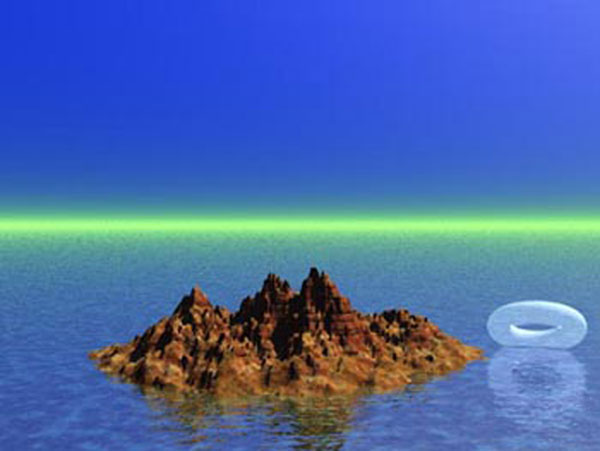This picture was created by Shahn Majid. It appears on his visions of noncommutative geometry page as well as in an extremely readable Plus-magazine article on Quantum geometry, written by Marianne Freiberger, explaining Shahn’s ideas. For more information on this, read Shahn’s SpaceTime blog.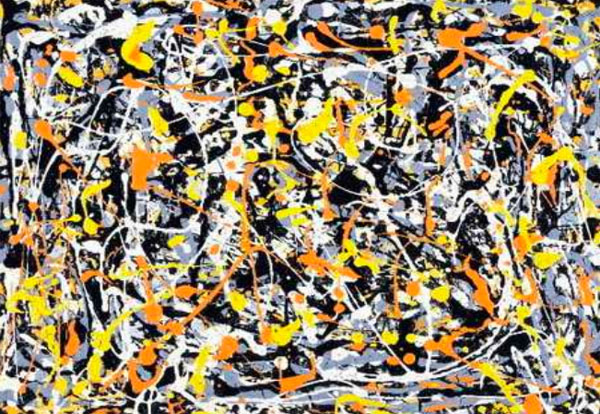This painting is Jackson Pollock‘s “Untitled N.3”. It depicts the way Matilde Marcolli imagines a noncommutative space. It is taken from her slides of her talk for a general audience Mathematicians look at particle physics.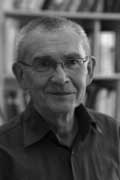Amidst all LHC-noise, Yuri I. Manin arXived today an interesting paper Cyclotomy and analytic geometry over $\mathbb{F}_1$.

The paper gives a nice survey of the existent literature and focusses on the crucial role of roots of unity in the algebraic geometry over the non-existent field with one element $\mathbb{F}_1$ (in French called ‘F-un’). I have tried to do a couple of posts on F-un some time ago but now realize, reading Manin’s paper, I may have given up way too soon…

At several places in the paper, Manin hints at a possible noncommutative geometry over $\mathbb{F}_1$ :

This is the appropriate place to stress that in a wider context of Toen-Vaqui ‘Au-dessous de Spec Z’, or eventually in noncommutative $\mathbb{F}_1$-geometry, teh spectrum of $\mathbb{F}_1$ loses its privileged position as a final object of a geometric category. For example, in noncommutative geometry, or in an appropriate category of stacks, the quotient of this spectrum modulo the trivial action of a group must lie below this spectrum.

Soule’s algebras $\mathcal{A}_X$ are a very important element of the structure, in particular, because they form a bridge to Arakelov geometry. Soule uses concrete choices of them in order to produce ‘just right’ supply of morphisms, without a priori constraining these choices formally. In this work, we use these algebras and their version also to pave a way to the analytic (and possibly non-commutative) geometry over $\mathbb{F}_1$.

Back when I was writing the first batch of F-un posts, I briefly contemplated the possibility of a noncommutative geometry over $\mathbb{F}_1$, but quickly forgot about it because I thought it would be forced to reduce to commutative geometry.

Here is the quick argument : noncommutative geometry is really the study of coalgebras (see for example my paper or if you prefer more trustworthy sources the Kontsevich-Soibelman paper). Now, unless I made a mistake, I think all coalgebras over $\mathbb{F}_1$ must be co-commutative (even group-like), so reducing to commutative geometry.

Surely, I’m missing something…

Yesterday, Yuri Manin and Matilde Marcolli arXived their paper
follow-up of their previous paper Continued fractions, modular symbols, and non-commutative geometry.
They motivate the title of the recent paper by :

In
[MaMar2](http://www.arxiv.org/abs/hep-th/0201036), these and similar
results were put in connection with the so called “holography”
principle in modern theoretical physics. According to this principle,
quantum field theory on a space may be faithfully reflected by an
appropriate theory on the boundary of this space. When this boundary,
rather than the interior, is interpreted as our observable
space‚Äìtime, one can proclaim that the ancient Plato’s cave metaphor
is resuscitated in this sophisticated guise. This metaphor motivated
the title of the present paper.

Here’s a layout of
Plato’s cave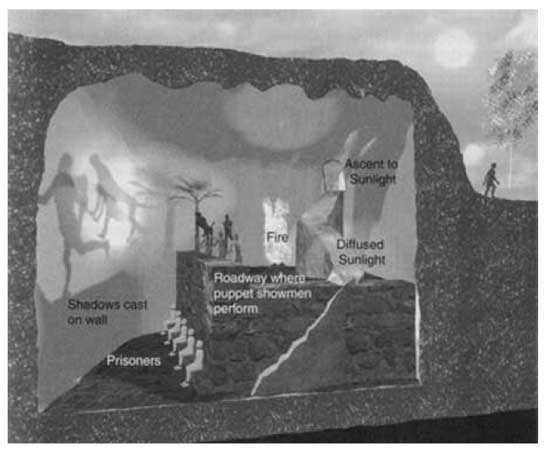Imagine prisoners, who have been chained since childhood deep inside an
cave: not only are their limbs immobilized by the chains; their heads
are chained as well, so that their gaze is fixed on a wall.
Behind
the prisoners is an enormous fire, and between the fire and the
prisoners is a raised walkway, along which statues of various animals,
plants, and other things are carried by people. The statues cast shadows
on the wall, and the prisoners watch these shadows. When one of the
statue-carriers speaks, an echo against the wall causes the prisoners to
believe that the words come from the shadows.
The prisoners
engage in what appears to us to be a game: naming the shapes as they
come by. This, however, is the only reality that they know, even though
they are seeing merely shadows of images. They are thus conditioned to
judge the quality of one another by their skill in quickly naming the
shapes and dislike those who begin to play poorly.
Suppose a
prisoner is released and compelled to stand up and turn around. At that
moment his eyes will be blinded by the firelight, and the shapes passing
will appear less real than their shadows.

Right, now how
does the Manin-Marcolli cave look? My best guess is : like this
picture, taken from Curt McMullen’s Gallery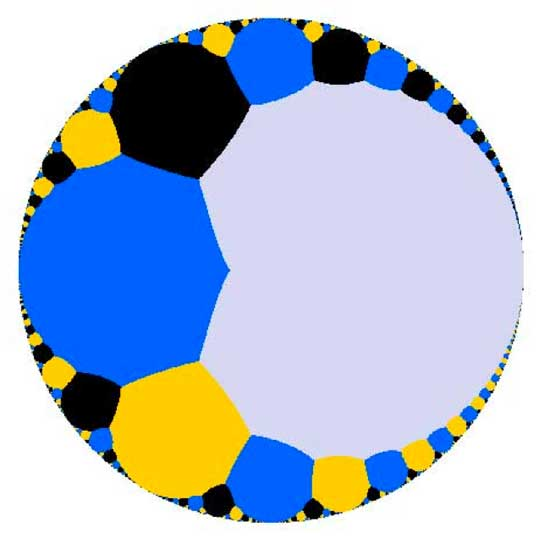Imagine
this as the top view of a spherical cave. M&M are imprisoned in the
cave, their heads chained preventing them from looking up and see the
ceiling (where $PSL_2(\mathbb{Z})$ (or a cofinite subgroup of
it) is acting on the upper-half plane via
Moebius-transformations ). All they can see is the circular exit of the
cave. They want to understand the complex picture going on over their
heads from the only things they can observe, that is the action of
(subgroups of) the modular group on the cave-exit
$\mathbb{P}^1(\mathbb{R})$. Now, the part of it consisting
of orbits of cusps
$\mathbb{P}^1(\mathbb{Q})$ has a nice algebraic geometric
description, but orbits of irrational points cannot be handled by
algebraic geometry as the action of $PSL_2(\mathbb{Z})$ is
highly non-discrete as illustrated by another picture from McMullen’s
gallerydepicting the ill behaved topology of the action on the bottom real
axis. Still, noncommutative _differential_ geometry is pretty good at
handling such ill behaved quotient spaces and it turns out that as a
noncommutative space, this quotient
$\mathbb{P}^1(\mathbb{R})/PSL_2(\mathbb{Z})$ is rich enough
to recover many important aspects of the classical theory of modular
curves. Hence, they reverse the usual NCG-picture of interpreting
commutative objects as shadows of noncommutative ones. They study the
$\mathbb{P}^1(\mathbb{R})/PSL_2(\mathbb{Z})$ of a classical
commutative object, the quotient of the action of the modular group (or
a cofinite subgroup of it) on the upper half-plane.

In our
noncommutative geometry course we have already
seen this noncommutative shadow in action (though at a very basic
level). Remember that we first described the group-structure of the
modular group $PSL_2(\mathbb{Z}) = C_2 \ast C_3$ via the
classical method of groups acting on trees. In particular, we
considered the tree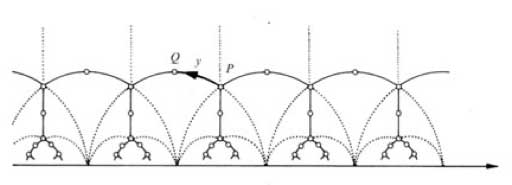and
calculated the stabilizers of the end points of its fundamental domain
(the thick circular edge). But
later we were able to give a
much shorter proof (due to Roger Alperin) by looking only at the action
of $PSL_2(\mathbb{Z})$ on the irrational real numbers (the
noncommutative shadow). Needless to say that the results obtained by
Manin and Marcolli from staring at their noncommutative shadow are a lot
more intriguing…

Here are
my nominees for the 2006 paper of the year award in mathematics &
mathematical physics : in math.RA : math.RA/0606241
: Notes on A-infinity
algebras, A-infinity categories and non-commutative geometry. I
by

Maxim Kontsevich
and
Yan Soibelman
. Here is the abstract :

We develop
geometric approach to A-infinity algebras and A-infinity categories
based on the notion of formal scheme in the category of graded vector
spaces. Geometric approach clarifies several questions, e.g. the notion
of homological unit or A-infinity structure on A-infinity functors. We
discuss Hochschild complexes of A-infinity algebras from geometric point
of view. The paper contains homological versions of the notions of
properness and smoothness of projective varieties as well as the
non-commutative version of Hodge-to-de Rham degeneration conjecture. We
also discuss a generalization of Deligne’s conjecture which includes
both Hochschild chains and cochains. We conclude the paper with the
description of an action of the PROP of singular chains of the
topological PROP of 2-dimensional surfaces on the Hochschild chain
complex of an A-infinity algebra with the scalar product (this action is
more or less equivalent to the structure of 2-dimensional Topological
Field Theory associated with an “abstract” Calabi-Yau
manifold).

why ? : Because this paper
probably gives the correct geometric object associated to a
non-commutative algebra (a huge coalgebra) and consequently the right
definition of a map between noncommutative affine schemes. In a previous post (and its predecessors) I’ve
tried to explain how this links up with my own interpretation and since
another time. in hep-th : hep-th/0611082 : Children’s Drawings From
Seiberg-Witten Curves
by Sujay K. Ashok, Freddy Cachazo, Eleonora
Dell’Aquila. Here is the abstract :

We consider N=2
supersymmetric gauge theories perturbed by tree level superpotential
terms near isolated singular points in the Coulomb moduli space. We
identify the Seiberg-Witten curve at these points with polynomial
equations used to construct what Grothendieck called “dessins
d’enfants” or “children’s drawings” on the Riemann
sphere. From a mathematical point of view, the dessins are important
because the absolute Galois group Gal(\bar{Q}/Q) acts faithfully on
them. We argue that the relation between the dessins and Seiberg-Witten
theory is useful because gauge theory criteria used to distinguish
branches of N=1 vacua can lead to mathematical invariants that help to
distinguish dessins belonging to different Galois orbits. For instance,
we show that the confinement index defined in hep-th/0301006 is a Galois
invariant. We further make some conjectures on the relation between
Grothendieck’s programme of classifying dessins into Galois orbits and
the physics problem of classifying phases of N=1 gauge theories.

why ? : Because this paper gives the
best introduction I’ve seen to Grothendieck’s dessins d’enfants
(slightly overdoing it by giving a crash course on elementary Galois
theory in appendix A) and kept me thinking about dessins and their
Galois invariants ever since (again, I’ll come back to this later).

Last time we
have seen that the _coalgebra of distributions_ of an affine smooth
variety is the direct sum (over all points) of the dual to the etale
local algebras which are all of the form $\mathbb{C}[[ x_1,\ldots,x_d ]]$ where $d$ is the dimension of the
variety. Generalizing this to _non-commutative_ manifolds, the first
questions are : “What is the analogon of the power-series algebra?” and
do all ‘points’ of our non-commutative manifold do have such local
algebras? Surely, we no longer expect the variables to commute, so a
non-commutative version of the power series algebra should be
$\mathbb{C} \langle \langle x_1,\ldots,x_d \rangle \rangle$,
the ring of formal power series in non-commuting variables. However,
there is still another way to add non-commutativity and that is to go
from an algebra to matrices over the algebra. So, in all we would expect
to be our _local algebras_ at points of our non-commutative manifold to
be isomorphic to $M_n(\mathbb{C} \langle \langle x_1,\ldots,x_d \rangle \rangle)$ As to the second question : _qurves_ (that is,
the coordinate rings of non-commutative manifolds) do have such algebras
as local rings provided we take as the ‘points’ of the non-commutative
variety the set of all _simple_ finite dimensional representations of
the qurve. This is a consequence of the _tubular neighborhood theorem_
due to [Cuntz](http://wwwmath.uni-muenster.de/u/cuntz/cuntz.html) and
[Quillen](http://www-history.mcs.st-andrews.ac.uk/Mathematicians/Quillen.html). In more details : If A is a qurve, then a simple
$n$-dimensional representation corresponds to an epimorphism
$\pi~:~A \rightarrow S = M_n(\mathbb{C})$ and if we take
$\mathfrak{m}=Ker(\pi)$, then
$M=\mathfrak{m}/\mathfrak{m}^2$ is an $S$-bimodule and
the $\mathfrak{m}$-adic completion of A is isomorphic to the
completed tensor-algebra $\hat{T}_S(M) \simeq M_n(\mathbb{C} \langle \langle x_1,\ldots,x_d \rangle \rangle)$ In contrast with
the commutative case however where the dimension remains constant over
all points, here the numbers n and d can change from simple to simple.
For n this is clear as it gives the dimension of the simple
representation, but also d changes (it is the local dimension of the
variety classifying simple representations of the same dimension). Here
an easy example : Consider the skew group algebra $A = \mathbb{C}[x] \star C_2$ with the action given by sending $x \mapsto -x$. Then A is a qurve and its center is
$\mathbb{C}[y]$ with $y=x^2$. Over any point $y \not= 0$ there is a unique simple 2-dimensional representation of A
giving the local algebra $M_2(\mathbb{C}[[y]])$. If
$y=0$ the situation is more complicated as the local structure
of A is given by the algebra $\begin{bmatrix} \mathbb{C}[[y]] & \mathbb{C}[[y]] \\ (y) & \mathbb{C}[[y]] \end{bmatrix}$ So, over
this point there are precisely 2 one-dimensional simple representations
corresponding to the maximal ideals $\mathfrak{m}_1 = \begin{bmatrix} (y) & \mathbb{C}[[y]] \\ (y) & \mathbb{C}[[y]] \end{bmatrix}~\qquad \text{and}~\qquad \mathfrak{m}_2 = \begin{bmatrix} \mathbb{C}[[y]] & \mathbb{C}[[y]] \\ (y) & (y) \end{bmatrix}$ and
both ideals are idempotent, that is $\mathfrak{m}_i^2 = \mathfrak{m}_i$ whence the corresponding bimodule $M_i = 0$ so the local algebra in either of these two points is just
$\mathbb{C}$. Ok, so the comleted local algebra at each point
is of the form $M_n(\mathbb{C}\langle \langle x_1,\ldots,x_d \rangle \rangle)$, but what is the corresponding dual coalgebra. Well,
$\mathbb{C} \langle \langle x_1,\ldots,x_d \rangle \rangle$ is
the algebra dual to the _cofree coalgebra_ on $V = \mathbb{C} x_1 + \ldots + \mathbb{C}x_d$. As a vectorspace this is the
tensor-algebra $T(V) = \mathbb{C} \langle x_1,\ldots,x_d \rangle$ with the coalgebra structure induced by the bialgebra
structure defined by taking all varaibales to be primitives, that is
$\Delta(x_i) = x_i \otimes 1 + 1 \otimes x_i$. That is, the
coproduct on a monomial gives all different expressions $m_1 \otimes m_2$ such that $m_1m_2 = m$. For example,
$\Delta(x_1x_2) = x_1x_2 \otimes 1 + x_1 \otimes x_2 + 1 \otimes x_1x_2$. On the other hand, the dual coalgebra of
$M_n(\mathbb{C})$ is the _matrix coalgebra_ which is the
$n^2$-dimensional vectorspace $\mathbb{C}e_{11} + \ldots + \mathbb{C}e_{nn}$ with comultiplication $\Delta(e_{ij}) = \sum_k e_{ik} \otimes e_{kj}$ The coalgebra corresponding to the
local algebra $M_n(\mathbb{C}\langle \langle x_1,\ldots,x_d \rangle \rangle)$ is then the tensor-coalgebra of the matrix coalgebra and
the cofree coalgebra. Having obtained the coalgebra at each point
(=simple representation) of our noncommutative manifold one might think
that the _coalgebra of non-commutative distributions_ should be the
direct sum of all this coalgebras, summed over all points, as in the
commutative case. But then we would forget about a major difference
between the commutative and the non-commutative world : distinct simples
can have non-trivial extensions! The mental picture one might have
about simples having non-trivial extensions is that these points lie
‘infinitesimally close’ together. In the $\mathbb{C}[x] \star C_2$ example above, the two one-dimensional simples have
non-trivial extensions so they should be thought of as a cluster of two
infinitesimally close points corresponding to the point $y=0$
(that is, this commutative points splits into two non-commutative
points). Btw. this is the reason why non-commutative algebras can be
used to resolve commutative singularities (excessive tangents can be
split over several non-commutative points). While this is still pretty
harmless when the algebra is finite over its center (as in the above
example where only the two one-dimensionals have extensions), the
situation becomes weird over general qurves as ‘usually’ distinct
simples have non-trivial extensions. For example, for the free algebra
$\mathbb{C}\langle x,y \rangle$ this is true for all simples…
So, if we want to continue using this image of points lying closely
together this immediately means that non-commutative ‘affine’ manifolds
behave like compact ones (in fact, it turns out to be pretty difficult
to ‘glue’ together qurves into ‘bigger’ non-commutative manifolds, apart
from the quiver examples of [this old
paper](http://www.arxiv.org/abs/math.AG/9907136)). So, how to bring
this new information into our coalgebra of distributions? Well, let’s
repeat the previous argument not with just one point but with a set of
finitely many points. Then we have a _semi-simple algebra_ quotient
$\pi~:~A \rightarrow S = M_{n_1}(\mathbb{C}) \oplus \ldots \oplus M_{n_k}(\mathb{C})$ and taking again
$\mathfrak{m}=Ker(\pi)$ and
$M=\mathfrak{m}/\mathfrak{m}^2$, then $M$ is again an
S-bimodule. Now, any S-bimodule can be encoded into a _quiver_ Q on k
points, the number of arrows from vertex i to vertex j being the number
of components in M of the form $M_{n_i \times n_j}(\mathbb{C})$. Again, it follows from the tubular neighborhood
theorem that the $\mathfrak{m}$-adic completion of A is
isomorphic to the completion of an algebra Morita equivalent to the
_path algebra_ $\mathbb{C} Q$ (being the tensor algebra
$T_S(M)$). As all the local algebras of the points are
quotients of this quiver-like completion, on the coalgebra level our
local coalgebras will be sub coalgebras of the coalgebra which is
co-Morita equivalent (and believe it or not but coalgebraists have a
name for this : _Takeuchi equivalence_) to the _quiver coalgebra_ which
is the vectorspace of the path algebra $\mathbb{C} Q$ with
multiplication induced by making all arrows from i to j skew-primitives,
that is, $\Delta(a) = e_i \otimes a + a \otimes e_j$ where the
$e_i$ are group-likes corresponding to the vertices. If all of
ths is a bit too much co to take in at once, I suggest the paper by Bill
Chin [A brief introduction to coalgebra representation
theory](http://condor.depaul.edu/~wchin/crt.pdf#search=%22%22A%20brief%20introduction%20to%20coalgebra%20representation%20theory%22%22). The
_coalgebra of noncommutative distributions_ we are after at is now the
union of all these Takeuchi-equivalent quiver coalgebras. In easy
examples such as the $\mathbb{C}[x] \star C_2$-example this
coalgebra is still pretty small (the sum of the local coalgebras
corresponding to the local algebras $M_2(\mathbb{C}[[x]])$
summed over all points $y \not= 0$ summed with the quiver
coalgebra of the quiver $\xymatrix{\vtx{} \ar@/^/[rr] & & \vtx{} \ar@/^/[ll]}$ In general though this is a huge object and we would
like to have a recipe to construct it from a manageable _blue-print_ and
that is what we will do next time.

Now
that my non-geometry
post
string-coffee-table post
which in turn is available through a
paper

The little
this : if you have a situation where a discrete group is acting with a
bad orbit-space (for example, $GL_2(\mathbb{Z})$ acting on the whole
complex-plane, rather than just the upper half plane) you can associate
to this a $C^*$-algebra and study invariants of it and interprete them
example is the one I mentioned and where the additional noncommutative
points (coming from the orbits on the real axis) seem to contain a lot
of modular information as clarified by work of Manin&Marcolli and
Zagier. Probably the best introduction into Connes-style
non-commutative geometry
from this perspective are the Lecture on
Arithmetic Noncommutative Geometry
by Matilde Marcolli. To
algebraists : this trick is very similar to looking at the
skew-group algebra $\mathbb{C}[x_1,\ldots,x_n] * G$ if
you want to study the _orbifold_ for a finite group action on affine
space. But as algebraist we have to stick to affine varieties and
polynomials so we can only deal with the case of a finite group,
analysts can be sloppier in their functions, so they can also do
something when the group is infinite.

By the way, the
skew-group algebra idea is also why non-commutative algebraic
geometry
enters string-theory via the link with orbifolds. The
easiest (and best understood) example is that of Kleinian singularities.
The best introduction to this idea is via the Representations
of quivers, preprojective algebras and deformations of quotient
singularities
notes by Bill Crawley-Boevey.

Artin-style non-commutative geometry aka
non-commutative projective geometry originated from the
work of Artin-Tate-Van den Bergh (in the west) and Odeskii-Feigin (in
the east) to understand Sklyanin algebras associated to elliptic curves
and automorphisms via ‘geometric’ objects such as point- (and
fat-point-) modules, line-modules and the like. An excellent survey
paper on low dimensional non-commutative projective geometry is Non-commutative curves and surfaces by Toby
Stafford and
Michel Van den Bergh
. The best introduction is the (also
neverending…) book-project Non-
commutative algebraic geometry
by Paul Smith who
maintains a
noncommutative geometry and algebra resource page
page (which is

Non-geometry
started with the seminal paper ‘Algebra extensions and
nonsingularity’, J. Amer. Math. Soc. 8 (1995), 251-289 by Joachim
Cuntz
and Daniel Quillen but which is not available online. An
online introduction is Noncommutative smooth
spaces
by Kontsevich and Rosenberg. Surely, different people have
different motivations to study non-geometry. I assume Cuntz got
interested because inductive limits of separable algebras are quasi-free
(aka formally smooth aka qurves). Kontsevich and Soibelman want to study
morphisms and deformations of $A_{\infty}$-categories as they explain in
their recent
paper
. My own motivation to be interested in non-geometry is the
hope that in the next decades one will discover new exciting connections
between finite groups, algebraic curves and arithmetic groups (monstrous
moonshine
being the first, and still not entirely understood,
instance of this). Part of the problem is that these three topics seem
to be quite different, yet by taking group-algebras of finite or
arithmetic groups and coordinate rings of affine smooth curves they all
turn out to be quasi-free algebras, so perhaps non-geometry is the
unifying theory behind these seemingly unrelated topics.

Here’s
an appeal to the few people working in Cuntz-Quillen-Kontsevich-whoever
noncommutative geometry (the one where smooth affine varieties
correspond to quasi-free or formally smooth algebras) : let’s rename our
topic and call it non-geometry. I didn’t come up with
this term, I heard in from Maxim Kontsevich in a talk he gave a couple
of years ago in Antwerp. There are some good reasons for this name
change.

The term _non-commutative geometry_ is already taken by
much more popular subjects such as _Connes-style noncommutative
differential geometry_ and _Artin-style noncommutative algebraic
geometry_. Renaming our topic we no longer have to include footnotes
(such as the one in the recent Kontsevich-Soibelman
paper
) :

We use “formal” non-commutative geometry
in tensor categories, which is different from the non-commutative
geometry in the sense of Alain Connes.

or to make a
distinction between _noncommutative geometry in the small_ (which is
Artin-style) and _noncommutative geometry in the large_ (which in
non-geometry) as in the Ginzburg notes.

Besides, the stress in _non-commutative geometry_ (both in Connes-
and Artin-style) in on _commutative_. Connes-style might also be called
‘K-theory of $C^*$-algebras’ and they use the topological
information of K-theoretic terms in the commutative case as guidance to
speak about geometrical terms in the nocommutative case. Similarly,
Artin-style might be called ‘graded homological algebra’ and they
use Serre’s homological interpretation of commutative geometry to define
similar concepts for noncommutative algebras. Hence, non-commutative
geometry is that sort of non-geometry which is almost
commutative…

But the main point of naming our subject
non-geometry is to remind us not to rely too heavily on our
(commutative) geometric intuition. For example, we would expect a
manifold to have a fixed dimension. One way to define the dimension is
as the trancendence degree of the functionfield. However, from the work
of Paul Cohn (I learned about it through Aidan Schofield) we know that
quasi-free algebras usually do’nt have a specific function ring of
fractions, rather they have infinitely many good candidates for it and
these candidates may look pretty unrelated. So, at best we can define a
_local dimension_ of a noncommutative manifold at a point, say given by
a simple representation. It follows from the Cunz-Quillen tubular
neighborhood result that the local ring in such a point is of the
form

$M_n(\mathbb{C} \langle \langle z_1,\ldots,z_m \rangle \rangle)$

(this s a noncommutative version of the classical fact
than the local ring in a point of a d-dimensional manifold is formal
power series $\mathbb{C} [[ z_1,\ldots,z_d ]]$) but in non-geometry both
m (the _local_ dimension) and n (the dimension of the simple
representation) vary from point to point. Still, one can attach to the
quasi-free algebra A a finite amount of data (in fact, a _finite_ quiver
and dimension vector) containing enough information to compute the (n,m)
couples for _all_ simple points (follows from the one quiver to rule them
all paper
or see this for more
details).

In fact, one can even extend this to points
corresponding to semi-simple representations in which case one has to
replace the matrix-ring above by a ring Morita equivalent to the
completion of the path algebra of a finite quiver, the _local quiver_ at
the point (which can also be computer from the one-quiver of A. The
local coalgebras of distributions at such points of
Kontsevich&Soibelman are just the dual coalgebras of these local
algebras (in math.RA/0606241 they
merely deal with the n=1 case but no doubt the general case will appear
in the second part of their paper).

The case of the semi-simple
point illustrates another major difference between commutative geometry
and non-geometry, whereas commutative simples only have self-extensions
(so the distribution coalgebra is just the direct sum of all the local
distributions) noncommutative simples usually have plenty of
non-isomorphic simples with which they have extensions, so to get at the
global distribution coalgebra of A one cannot simply add the locals but
have to embed them in more involved coalgebras.

The way to do it
is somewhat concealed in the
third version of my neverending book
(the version that most people
found incomprehensible). Here is the idea : construct a huge uncountable
quiver by taking as its vertices the isomorphism classes of all simple
A-representations and with as many arrows between the simple vertices S
and T as the dimension of the ext-group between these simples (and
again, these dimensions follow from the knowledge of the one-quiver of
A). Then, the global coalgebra of distributions of A is the limit over
all cotensor coalgebras corresponding to finite subquivers). Maybe I’ll
revamp this old material in connection with the Kontsevich&Soibelman
paper(s) for the mini-course I’m supposed to give in september.